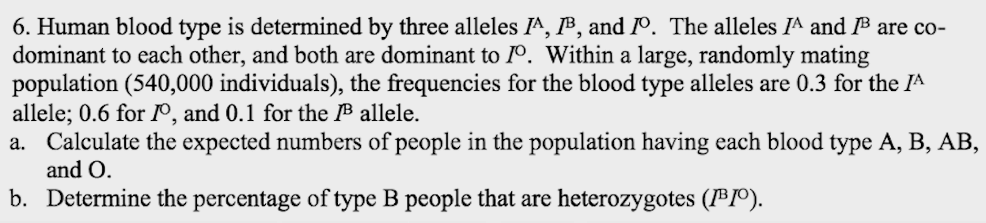# Problem: Human blood type is determined by three alleles, IA, IB, and IO. The alleles IA and IB are codominant to each other, and both are dominant to IO. Within a large, randomly mating population (540,000 individuals), the frequencies for the blood type alleles are 0.3 for the IA allele; 0.6 for the IO, and 0.1 for the IB allele.a. Calculate the expected number of people in the population having each blood type A, B, AB, and O.b. Determine the percentage of type B people that are heterozygous.

###### FREE Expert Solution

With the assumption that the population follows Hardy-Weinberg equilibrium, it can be expected that it follows the equation p2 + 2pq + q2 + 2qr + r2 + 2pr = 1, where p is the allele frequency for IA, q for IB, and r for IO

The other terms are defined below, along with their values:

p(0.09) is for the genotypic frequency for homozygous IAIA

2pq (0.36) is for heterozygous IAIO

q2 (0.36) is for homozygous IOIO

2qr (0.12) is for heterozygous IBIO

r2 (0.01) is for homozygous IBIB, and

2pr (0.06) is for heterozygous IAIB

It should be noted that unlike systems with just two alleles, having three alleles would modify the other equation to p + q+ r = 1.###### Problem Details

Human blood type is determined by three alleles, IA, IB, and IO. The alleles IA and IB are codominant to each other, and both are dominant to IO. Within a large, randomly mating population (540,000 individuals), the frequencies for the blood type alleles are 0.3 for the IA allele; 0.6 for the IO, and 0.1 for the IB allele.

a. Calculate the expected number of people in the population having each blood type A, B, AB, and O.

b. Determine the percentage of type B people that are heterozygous.